Mathematical and Physical Journal
for High Schools
Issued by the MATFUND Foundation
 Already signed up? New to KöMaL?

#Problem I. 259. (February 2011)

I. 259. Recursion is a tool often used both in mathematics and in informatics. It can appear in different stages during the solution of a problem, for example, in the specification part, in algorithmic planning, or during the coding in the programming language.

One typically uses a function to give the specification. This function effectively establishes a connection between the input and the output. Let us first consider the power function for example. A possible definition is the following: $\displaystyle x^{n}=x\cdot x^{n-1}$ and $\displaystyle x^{0}=1$, or, in other words,

$\displaystyle \mathop{\rm power}\, (x,n)= \begin{cases} 1 & \text{if \ } n=0, \\ x \cdot \mathop{\rm power}\, (x,n-1) & \text{if \ } n>0. \end{cases}$

In this way, the computation of $\displaystyle 5^{3}$ is reduced to $\displaystyle 5\cdot 5^{2}$, that is to $\displaystyle 5^{2}$, then to $\displaystyle 5^{1}$, finally, to $\displaystyle 5^{0}$, which is defined separately. The above task can be solved effectively with a recursive algorithm: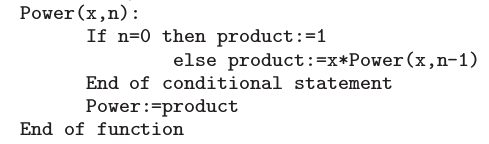In functional programming languages, like ImagineLogo, coding simply follows from the above: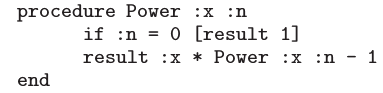Non-recursive languages, like Pascal, may also allow this coding to be implemented: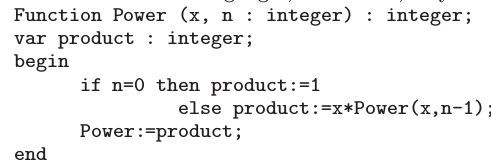Algorithms and programs defined iteratively can be rewritten in a recursive fashion. Sequences and conditionals, as the most important algorithmic blocks, can be transformed without any difficulty or modification. Loops however require some revision to make them recursive. The following scheme illustrates how to reformulate a pre-test loop (that is, a loop in which the loop test is evaluated before the body of the loop):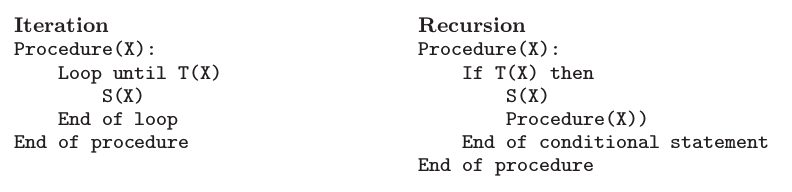Example. Create the sequence of natural numbers between $\displaystyle A$ and $\displaystyle B$ ($\displaystyle 1\le A,B\le 100$). In the solution below, the procedure Előállít(5, 10, Sor, 1) puts the sequence 5 6 7 8 9 10 into the array Sor.

I. Iterative solution: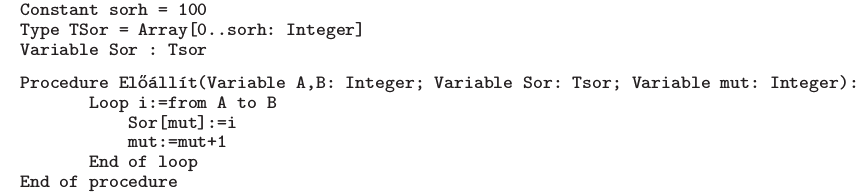II. Recursive solution: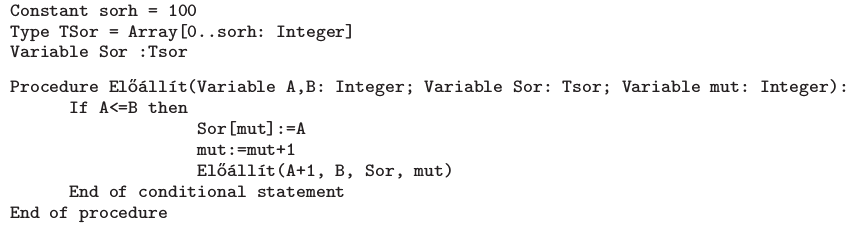Your task is to create a program that produces the following sequences without using any loop.

$\displaystyle a)$ Write a function to create a mountain of numbers'' of height $\displaystyle N$ ($\displaystyle N\ge 1$). Example: mountain 5 Result: 1 2 3 4 5 4 3 2 1;

$\displaystyle b)$ Write a function to create a mountain chain of numbers'' of height $\displaystyle N$. Example: chain 4 Result: 1 1 2 1 1 2 3 2 1 1 2 3 4 3 2 1;

$\displaystyle c)$ Write a function to create a staircase of numbers'' of height $\displaystyle N$. Example: staircase 6 Result: 1 2 2 3 3 3 4 4 4 4 5 5 5 5 5 6 6 6 6 6 6;

$\displaystyle d)$ Write the first $\displaystyle N$ elements of the following recursive sequence: $\displaystyle a_{1}=-1$ and $\displaystyle a_{n}=-4 - 3a_{n-1}$.

The first argument to your program is the value of $\displaystyle N$, while the output file is specified by the second argument. The output file should contain one sequence in each line. Elements of a sequence should be separated by a space. You should not use loops when writing into the file.

The source code (i259.pas, i259.cpp, ...) together with a short documentation (i259.txt, i259.pdf, ...) -- also describing which developer environment to use for compiling, further a brief description of your solution -- should be submitted.

(10 pont)

Deadline expired on March 10, 2011.

Sorry, the solution is available only in Hungarian. Google translation

Mintamegoldásként Szabó Attila (Pécs, Leőwey Klára Gimnázium, 10. osztály) tanuló munkáját közöljük: i259.pas

### Statistics:

 11 students sent a solution. 10 points: Gema Barnabás, Hoffmann Áron, Kalló Kristóf, Seres Márk Dániel, Szabó 928 Attila. 9 points: Barta 111 János, Kocsis 789 Mátyás, Leitereg András, Sztanka-Tóth Tamás, Varga 256 Erik. 7 points: 1 student.

Problems in Information Technology of KöMaL, February 2011